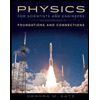# A container with volume 1.63 L is initially evacuated. Then it is filled with 0.226 g of N2. Assume that the pressure of the gas is low enough for the gas to obey the ideal-gas law to a high degree of accuracy.If the root-mean-square speed of the gas molecules is 192 m/s , what is the pressure of the gas? Express the answer in pascals.

Question
100%

A container with volume 1.63 L is initially evacuated. Then it is filled with 0.226 g of N2. Assume that the pressure of the gas is low enough for the gas to obey the ideal-gas law to a high degree of accuracy.If the root-mean-square speed of the gas molecules is 192 m/s , what is the pressure of the gas? Express the answer in pascals.

Expert Solution

### Want to see the full answer?

Check out a sample Q&A hereStudents who’ve seen this question also like:Physics for Scientists and Engineers: Foundations and Connections
1st Edition
ISBN: 9781133939146
Author: Katz, Debora M.
Publisher: Cengage Learning